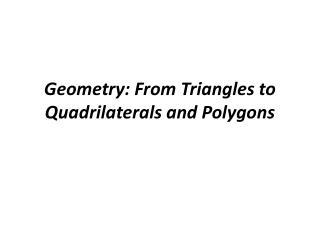DownloadDownload PresentationGeometry: From Triangles to Quadrilaterals and Polygons

Geometry: From Triangles to Quadrilaterals and Polygons

Télécharger la présentationGeometry: From Triangles to Quadrilaterals and Polygons

- - - - - - - - - - - - - - - - - - - - - - - - - - - E N D - - - - - - - - - - - - - - - - - - - - - - - - - - -
Presentation Transcript

1. Geometry: From Triangles to Quadrilaterals and Polygons

2. MA.912.G.3.2 Compare and contrast special quadrilaterals on the basis of their properties. Block 24

3. Convex quadrilaterals are classified as follows: • Trapezoid (Amer.): exactly one pair of opposite sides is parallel. • Parallelogram: both pairs of opposite sides are parallel.

4. Convex quadrilaterals are classified as follows: • Rhomb (Rhombus): all four sides are of equal length • Kite: two adjacent sides are of equal length and the other two sides also of equal length

5. Convex quadrilaterals are further classified as follows: • Rectangle: all four angles are right angles • Square: all four sides are of equal length and all four angles are equal (equiangular), with each angle a right angle.

8. Parallelograms Parallelogram: both pairs of opposite sides are parallel.

9. Test for Parallelograms Parallelogram: both pairs of opposite sides are parallel. In addition to that definition we have tests to determine if a quadrilateral is parallelogram like the following:

10. Test for Parallelograms The quadrilateral is a parallelogram: • If the opposite sides of a quadrilateral are congruent

11. Test for Parallelograms The quadrilateral is a parallelogram: • If both pairs of opposite angles of a quadrilateral are congruent

12. Test for Parallelograms The quadrilateral is a parallelogram: • If the diagonals of a quadrilateral bisect each other

13. Test for Parallelograms The quadrilateral is a parallelogram: • If one pair of opposite sides is parallel and congruent

14. Kite • Kite: two adjacent sides are of equal length and the other two sides also of equal length. • This implies that one set of opposite angles is equal, and that one diagonal perpendicularly bisects the other.

15. Rhomb : • Rhomb: all four sides are of equal length. • This implies that opposite sides are parallel, opposite angles are equal, and the diagonals perpendicularly bisect each other.

16. Rectangle • Rectangle (or Oblong): all four angles are right angles. • This implies that opposite sides are parallel and of equal length, and the diagonals bisect each other and are equal in length.

17. Square Square (regular quadrilateral): all four sides are of equal length (equilateral), and all four angles are equal (equiangular), with each angle a right angle. This implies that opposite sides are parallel (a square is a parallelogram), and that the diagonals perpendicularly bisect each other and are of equal length. A quadrilateral is a square if and only if it is both a rhombus and a rectangle.

18. Trapezoid: • Trapezoid (Amer.): exactly one pair of opposite sides is parallel. • The parallel sides are called bases, and the nonparallel sides are called legs • If the legs are congruent then the trapezoid is called isosceles trapezoid.

19. Test for Parallelograms and Coordinates If the quadrilateral is graphed on the coordinate plane you can use Distance Formula, Slope Formula and Midpoint Formula. The Slope Formula is used to determine if the opposite sides are parallel the Distance Formula is used to test opposite sides for congruency, the Midpoint Formula can be used to determine if the diagonals are bisecting each other

20. Example: • We will check if a given quadrilateral on the coordinate plane is a parallelogram

21. Question: Prove that quadrilateral ABCD where • A= (-1,-1) • B=(3,0) • C=(4,2) • D=(0,1) • Is a parallelogram

22. Proof: We will prove that AB||CD and AD||BC (opposite sides of quadrilateral are parallel) We will use the slope formula:

23. Proof: We will prove that AB||CD and AD||BC We will use the slope formula:

24. Proof: We will prove that AB||CD and AD||BC We will use the slope formula:

25. Proof: We proved that both pairs of slopes are the same so: AB||CD and AD||BC hence the quadrilateral ABCD is a parallelogram

26. Now answer questions from the handout • Find the diagonals of the parallelogram • Find the intersection point of the diagonals • Verify that the diagonals bisect each other

27. Review and discussion • Create the Venn diagram and ,,family tree” of quadrilaterals • Discus properties of quadrilaterals